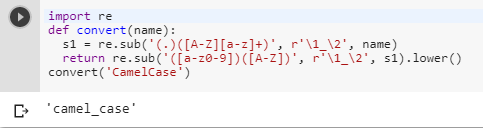2 views
in Python

Example:

>>> convert('CamelCase')

'camel_case'

by (106k points)

There are many ways of converting Python function into CamelCase to snake_case some important ways are as follows:-

While doing these type of conversion questions regular expressions are the best way to go with below is the code that shows how we can convert CamelCase to snake_case:-

import re

def convert(name):

s1 = re.sub('(.)([A-Z][a-z]+)', r'\1_\2', name)

return re.sub('([a-z0-9])([A-Z])', r'\1_\2', s1).lower()

convert('CamelCase')If you feel the regular expression a bit tougher and complex to use than you can use the inflection library which is in the package index that can handle these things for you. In this case, you'd be looking for inflection.underscore() function:

inflection.underscore('CamelCase')